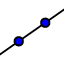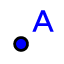# Angles and Demons

Topic:
Angles

## Definition 8-12

A plane angle is the inclination to one another of two lines in a plane which meet one another and do not lie in a straight line. Just an angle, we know what they are but we need a definition that builds from our previous terms. This is saying an angle (in a plane, remember, always in a plane for us) is a measure of the gap between to intersecting lines. There are many ways to measure angles, the two main forms are degrees and radians. Degrees are an arbitrary (picked by personal preference) unit of measure for angles that defines a circle to contain 360o for the simple reason that 360 is a biggish number with a lot of factors; lots of factors means nicer fractions. Good news, we stick with degrees when using geometry for the most part. Radians are not arbitrary, they define a complete rotation around a circle to be 2 because the circumference of a circle with a radius of 1 would be 2; radians are just telling you the amount of the circle's circumference you have rotated through. For example, if you rotate a 1/4 of the way around the circle then you have covered a 1/4 of the circle's circumference and the measure of that angle is (). Once you get used to radians, they are a much more useful system in higher level math. And when the lines containing the angle are straight, the angle is called rectilinear. When the sides of an angle make a straight line, then the angle is called rectilinear; literally "straight line" in Latin. As simple as that, we don't really use this term much but we do know that these angles are 1800 When a straight line standing on a straight line makes the adjacent angles equal to one another, each of the equal angles is right, and the straight line standing on the other is called perpendicular to that on which it stands. How would you define right angles? 900? A quarter of a circle? It's hard to define right without angle measures, but remember that Euclid didn't measure. All right angles really are are the angles formed when lines are perpendicular and lines are perpendicular when all the angles they form when they intersect are equal. That's actually a really easy way to define right that doesn't use the idea of degrees. Perpendicular comes from a latin phrase that means "to hang through" (per- through, pendere-to hang; pendulum comes from this word too). Imagine taking a string with a weight tied to the end of it, it will hang straight down, if you hold this string up to the horizon you will make a right angle with the horizon. An obtuse angle is an angle greater than a right angle. We are used to this. Obtuse comes from a Latin word that means to beat against or to blunt something (as in to make a knife dull and blunt). If you looked at an obtuse angle as the edge of a knife with would be blunt (not sharp). An acute angle is an angle less than a right angle. Easy again. Acute comes from a Latin word that means sharp, if you looked at an acute angle as the edge of a knife it would be sharp; old English also used acute to mean small, which is why we say small animals are cute.

## Practice with the tools

Use the tools in the applet to draw angles. Though Euclid didn't measure, we will because it can help convince us sometimes that what we are doing is correct. We have a new toolnow that lets us measure angles. Draw a set of intersecting lines using,, orthen use theto add a point by clicking on the intersection of the two lines. We now should see two types of points, blue points and grey points. Blue points are independent and you can move them while grey points are dependent on something else and can only be moved if the connected items are moved. If you are using the standard point labels, you can move A, B, C, and D by using, then clicking and dragging them. You cannot do this with E because it is dependent on the lines and you can only move E by moving the lines instead. Lets measure an angle, clickthen click on the points C, E, A in that order. appears and tells us the degree measure of angle CEA, if you useand drag the blue points around then you will see change accordingly. Play around with thetool on the different angles, try measuring the same angle again but switch the order of the letters and see if anything changes.